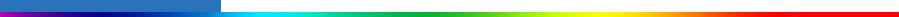Working Group I: The Scientific BasisGet Javascript Other reports in this collection

 Appendix 12.3: Pattern Correlation Methods The pattern correlation methods discussed in this section are closely related to optimal detection with one signal pattern. Pattern correlation studies use either a centred statistic, R, which correlates observed and signal anomalies in space relative to their respective spatial means, or an uncentred statistic, C (Barnett and Schlesinger, 1987), that correlates these fields without removing the spatial means. It has been argued that the latter is better suited for detection, because it includes the response in the global mean, while the former is more appropriate for attribution because it better measures the similarity between spatial patterns. The similarity between the statistics is emphasised by the fact that they can be given similar matrix-vector representations. In the one pattern case, the optimal (regression) estimate of signal amplitude is given by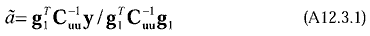The uncentred statistics may be written similarly as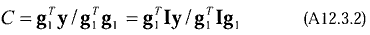where I is the n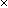n identity matrix. Similarly, the centred statistic can be written (albeit with an extra term in the denominator) as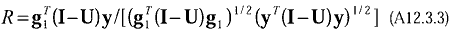where U is the nn matrix with elements ui,j=1/n. The matrix U removes the spatial means. Note that area, mass or volume weighting, as appropriate, is easily incorporated into these expressions. The main point is that each statistic is proportional to the inner product with respect to a matrix “kernel” between the signal pattern and the observations (Stephenson, 1997). In contrast with the pattern correlation statistics, the optimal signal amplitude estimate, which is proportional to a correlation coefficient using the so-called Mahalonobis kernel (Stephenson, 1997), maximises the signal-to-noise ratio.